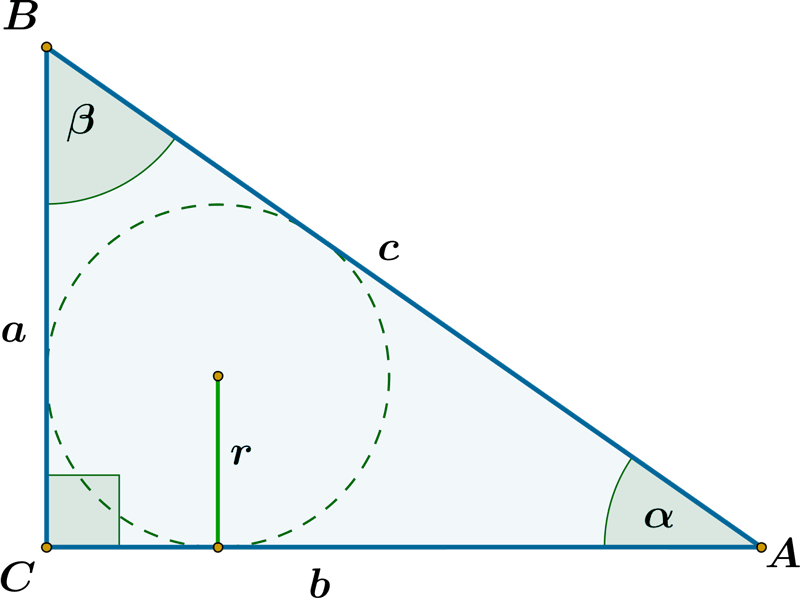Pythagorean theorem calculator

# The Ultimate Tool for Solving Right Triangles

A right triangle is a triangle with one angle measuring 90 degrees. Solving right triangles is important in geometry. It involves finding missing sides or angles. It is useful in various fields such as construction, navigation, and engineering.

## Basic Concepts in Right Triangles

• The Pythagorean Theorem states that in a right triangle. The square of the hypotenuse is equal to the sum of the squares of the other two sides.
• The sides of a right triangle are often referred to as the opposite, adjacent, and hypotenuse.
• Trigonometric ratios such as sine, cosine, and tangent. They are used to relate the sides and angles of a right triangle.

## Using Trigonometric Ratios to Solve Right Triangles

To use trigonometric ratios to solve right triangles. You need to identify the angle and the side you want to solve for. And then use the appropriate ratio to find the missing value by using Pythagorean Theorem Calculator.

## Special Right Triangles

• Special right triangles have specific angle measurements and ratios between their sides. That make them easier to solve.
• The 45-45-90 triangle has two congruent sides and the hypotenuse is the square root of 2 times the length of one of the legs.
• The 30-60-90 triangle has sides in the ratio of 1:√3:2 and one acute angle measures 30 degrees while the other measures 60 degrees.
• Examples of solving special right triangles using trigonometric ratios will be provided.

## The Ultimate Tool: Using the Law of Sines and Law of Cosines

• The Law of Sines and the Law of Cosines are used to solve any triangle (not just right triangles). It can be used to solve right triangles as well.
• The Law of Sines states that the ratio of the length of a side to the sine of its opposite angle is the same for all three sides of a triangle.
• The Law of Cosines can be used to find any side or angle of a triangle when you know the lengths of the other two sides and the included angle.
• Examples of solving right triangles using the Law of Sines and the Law of Cosines will be provided.

## Practical Applications of Solving Right Triangles

Solving right triangles has practical applications in various fields. Such as architecture, construction, navigation, and engineering.

For example, architects use right triangle trigonometry to calculate the height and length of staircases, and engineers use it to calculate the slope of roads and bridges.

Understanding right triangle trigonometry is important in everyday life as well. Such as measuring the height of a tree or the distance between two points by using the Pythagorean theorem calculator.

## Steps to solve a right triangle manually

Here are the steps to solve a right triangle manually:

### Identify the known values

Look at the given information and identify the values you know. This may include the length of one or more sides, one or more angles, or both.

### Identify the unknown values

Determine which values you need to solve for. This may include the length of one or more sides, one or more angles, or both.

### Use the Pythagorean Theorem to find missing sides

If you know the length of two sides of a right triangle. You can use the Pythagorean Theorem to find the length of the third side (the hypotenuse). The theorem states that a^2 + b^2 = c^2, where c is the hypotenuse and a and b are the other two sides.

### Use trigonometric ratios to find missing sides or angles

If you know the measure of an angle and the length of one side of a right triangle. You can use trigonometric ratios to find the length of another side. The three trigonometric ratios are sine, cosine, and tangent. And they relate the lengths of the sides of a right triangle to the measure of an angle.

The sine ratio is sin (theta) = opposite/hypotenuse.

The cosine ratio is cos (theta) = adjacent/hypotenuse.

The tangent ratio is tan (theta) = opposite/adjacent.

### Use special right triangles to find missing sides or angles

If the right triangle is a special right triangle. You can use its properties to find the lengths of the sides and the measure of the angles.

Use the Law of Sines or Law of Cosines to find missing sides or angles

## Steps to solve the right triangle by using a calculator

Here are the steps to solve a right triangle using the Pythagorean theorem Calculator:

• Identify the known values
• Identify the unknown values
• Choose the appropriate function on the calculator
• Enter the values into the calculator
• Solve for the unknown value
• Round the answer, if necessary

## Conclusion

Solving right triangles is an important skill in geometry. It has practical applications in various fields. The ultimate tool for solving right triangles involves using trigonometric ratios. Special right triangles, and the Law of Sines and Law of Cosines. Understanding right triangle trigonometry is beneficial for future studies and careers.